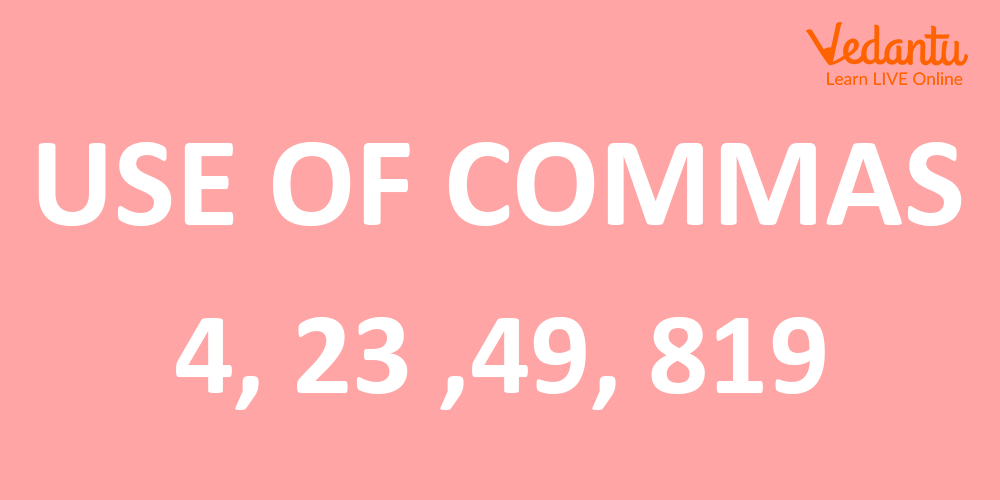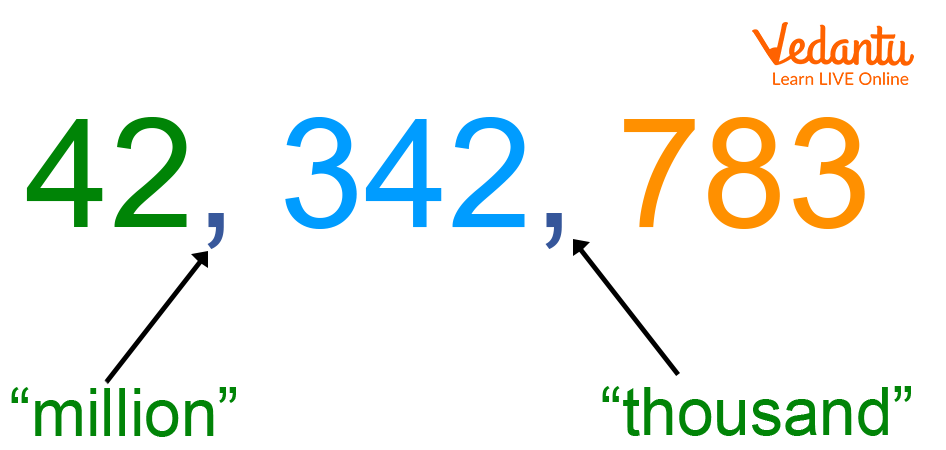Courses
Courses for Kids
Free study material
Free LIVE classes
More

# What are Numerals?LIVE
Join Vedantu’s FREE Mastercalss

## What are the Ways to Represent Numbers in Maths?

Are you looking for different ways to represent numbers? Here, numerals will help you to represent numbers using different ways.

In Maths, numerals are numbers, symbols, figures, or groups of symbols or figures to represent any given number. For example, If Tina has 6 chocolates, then she can represent this by writing the digit “6”, the word “six”, the roman numeral VI, or she can also hold up six of her fingers. There are many different ways to represent the number of chocolates she has. But, all these ways mean the same thing that Tina has 6 chocolates.

What are Digits in Numerals?

A digit is a single symbol used to represent numbers.

The 10 digits we use in everyday numerals include 1, 2, 3, 4, 5, 6, 7, 8, 9, and 10.

Example:

The numeral 172 is made up of three digits: 1, 7, and 2.

The numeral 53 is made up of two digits: 5 and 3.

The numeral 8 is made up of only one digit, i.e., 8. Hence, a single digit can also be a numeral.

Note: We can also use other symbols to represent numbers. For example, hexadecimal numbers used letters such as A, B, C, D, E, etc. to represent numbers like 10, 11, 12, 13, and 14, respectively.

### What is the Indian Numeral System?

The Indian numeral system is a writing system adapted in India for expressing numbers. Here, numbers are split into different periods or groups. This numeral system comprises a set of numerals formed by 10 different symbols such as 0, 1, 2, 3, 4, 5, 6, 7, 8, and 9.

When we use the Indian numeral system, the position of digits goes in a sequence of ones, tens, hundreds, thousands, ten thousand, lakhs, ten lakhs, crores, and ten crores. The below table represents the period and places according to the number of digits in a number.

 Periods Places Number of Digits Crores Ten Crores (TC)10,00,00,000 9 Crores (C)1,00,00,000 8 Lakhs Ten Lakhs (TL)10,00,000 7 Lakhs (L)1,00,000 6 Thousands Ten Thousands (T Th)10,000 5 Thousands (Th)1000 4 Ones Hundreds (H)100 3 Tens (T)10 2 Ones (0)1 1

### Use of Commas In Indian Numeral System

In the Indian numeral system, commas are used after every period to represent numbers in its standard numeral form. For example, the number 356789102 can be better represented as 35,67,89,102 in the standard numeral system using commas after every period. The number 35,67,89,102 can be read as thirty-five crores, sixty-seven lakhs, eight-nine thousand, one hundred, and two.Use of comma in the Indian Numeral System

### What is the International Numeral System?

The International numeral system is another method of representing numbers. In this system also, the numbers are split into different periods or groups which makes larger numbers easier to read.

When we use the International numeral system, the position of digits goes in a sequence of ones, tens, hundreds, thousands, ten thousands, hundred thousands, million, ten millions, and hundred millions. Look at the table below to understand how the International numeral system works.

 Periods Places Millions Hundred Millions (HM)100,000,000 Ten Millions (TM)10,000,000 Millions (M)1,000,000 Thousands Hundred Thousands (H TH)100,000 Ten Thousands (T Th)10,000 Thousands (Th)1000 Ones Hundreds (H)100 Tens (T)10 Ones (0)1

### Use of Commas in the International Numeral System

In this numeral system also, commas are used after every period to represent numbers in its standard numeral form. For example, the number 2567823 can be better represented as 2,567,823 in the standard numeral system using commas after every period. The number 2,567,823 can be read as two million five hundred sixty-seven thousand eight hundred and twenty three.Use of comma In International Numeral System

### What are the Different Types of Numerals?

The different types of numerals include the following.

Roman Numerals

Ancient Romans used special numerals to represent numbers. They used C to represent 100 and X to represent 10.

The Roman numerals are based on the following symbols.

 1 5 10 50 100 500 1000 I V X L C D M

The symbols can be combined to make other numbers like 2, 3, 4, 5, 6, and so on.

Decimal Numerals

Decimal numerals, also known as Hindu-Arabic Numeral System, Arabic Numeral System, and base-10 numerals system in Mathematics, use 10 different numerals such as 0, 1, 2, 3, 4, 5, 6, 7, 8, and 9 to represent numbers. Decimal numerals also require decimal points to represent a decimal fraction.

Example:

Thirty-five and 7 tenths is written as 35.7 in decimals.

Because

35.7 has 3 tens, 5 ones, and 7 tenths as shown below:

35.7 = 30 + 5 + 710

In decimal numerals, the numerals used in representing numbers take different place values depending on their positions.

Example:

In base 10 system, the number 342.65 represents the sum as ( 3 10²) + (4 10¹) + ( 2 10⁰) + ( 6 10⁻¹) + ( 5 10⁻²).

Binary Numerals

Binary numerals, also known as base-2 numeral systems, use only two symbols such as 0 and 1 to represent any value. Each digit or symbol is used in binary numerals to represent a number known as bit. For example, 110100 is a binary number.

There is no use of 2, 3, 4, 5, 6, 7, 8, and 9 in Binary.

Hexadecimal numeral, also known as a base-16 numeral system, uses 16 digits or symbols, to represent any number. The symbols or digits used in the hexadecimal system are 1, 2, 3, 4, 5, 6, 7, 8, 9, A, B, C, D, E, and F. Here A is 10, B is 11, and so on.

The place value in hexadecimal numerals is determined by the power of 16.

Example:

5A2₁₆ = 5 16² + 10 16¹ + 2 16⁰ = 1280 + 160 + 2 = 1442₁₀.

### Conclusion

In short, the numeral is a word, figure, symbol, or letter used to represent a number. There are different types of numerals to represent a given number. In this article, we have 5 types of numerals, but there are other different types of numerals as well.

Last updated date: 22nd Sep 2023
Total views: 138k
Views today: 4.38k

## FAQs on What are Numerals?

1. What is the simplest numeral system to represent natural numbers?

The simplest numeral system to represent natural numbers is the unary numeral system. In this system, the digit 0 is represented by the empty string or empty words whereas the natural numbers 1, 2, 3, 4, 5, 6, etc. are represented as 1, 11, 111, 1111, 11111, 111111, respectively.

2. What is the difference between numbers and numerals?

The primary difference between number and numeral is that the number is a concept whereas the numeral is a way to write numbers. A number can be represented in different ways using numerals. Therefore, each numeral will represent the same number under a specific number system.